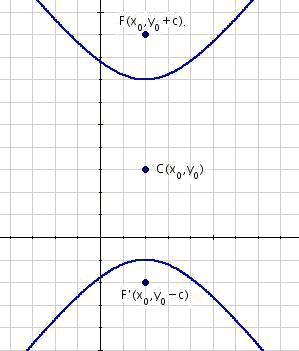# Equation of the vertical hyperbolasNext the vertical hyperbolas are analyzed with center in the generic point $$C(x_0,y_0)$$. The focal axis is now parallel to the ordinate axis, and therefore the foci are at points $$F'(x_0,y_0-c)$$ and $$F(x_0,y_0+c)$$.

Applying now the general definition we obtain $$\sqrt{(x-x_0)^2+(y-y_0+c)^2}-\sqrt{(x-x_0)^2+(y-y_0-c)^2}=2a$$$The root is added, and we square it: $$\begin{array}{rcl} \Big(\sqrt{(x-x_0)^2+(y-y_0+c)^2}\Big)^2 & = & \Big( 2a+\sqrt{(x-x_0)^2+(y-y_0-c)^2} \Big)^2 \\ (x-x_0)^2+(y-y_0+c)^2 & = & 4a^2+4a \sqrt{(x-x_0)^2+(y-y_0-c)^2}+ \\ & & +(x-x_0)^2+(y-y_0-c)^2 \\ (x-x_0)^2+(y-y_0)^2+2(y-y_0)c+c^2 & = & 4a^2+4a \sqrt{(x-x_0)^2+(y-y_0-c)^2}+ \\ & & +(x-x_0)^2+(y-y_0)^2-2(y-y_0)^2 \\ & & -2(y-y_0)c+c^2\end{array}$$$

On having simplified and dividing by four: $$\begin{array}{rcl} 4(y-y_0)c & = & 4a^2+4a\sqrt{(x-x_0)^2+(y-y_0-c)^2} \\ (y-y_0)c & = & a^2+a \sqrt{(x-x_0)^2+(y-y_0-c)^2} \end{array}$$$On having cleared the root and having squared again: $$\begin{array}{rcl} (c(y-y_0)-a^2)^2 & = & \Big(a\sqrt{(x-x_0)^2+(y-y_0-c)^2}\Big)^2 \\ c^2(y-y_0)^2-2a^2c(y-y_0)+a^4 & = & a^2((x-x_0)^2+(y-y_0-c)^2) \\ c^2(y-y_0)^2-2a^2c(y-y_0)+a^4 & = & a^2 ((x-x_0)^2+(y-y_0)^2-2c(y-y_0)+c^2) \\ c^2(y-y_0)^2-2a^2c(y-y_0)+a^4 & = & a^2(x-x_0)^2+a^2(y-y_0)^2-2a^2c(y-y_0)+a^2c^2 \\ c^2(y-y_0)^2-a^2(y-y_0)^2-a^2(x-x_0)^2& = & a^2 c^2-a^4 \\ (c^2-a^2)(y-y_0)^2-a^2(x-x_0)^2 & = & a^2(c^2-a^2) \end{array}$$$

Then divide by $$a^2(c^2-a^2)$$ to get $$1$$ on the right: $$\displaystyle \begin{array}{rcl} \frac{(c^2-a^2)(y-y_0)^2}{a^2(c^2-a^2)}-\frac{a^2(x-x_0)^2}{a^2(c^2-a^2)} & = & 1 \\ \frac{(y-y_0)^2}{a^2}-\frac{(x-x_0)^2}{(c^2-a^2)}=1 \end{array}$$$On having applied the definition $$c^2=a^2+b^2=$$, $$b^2= c^2-a^2$$ is replaced and we get the equation desired for the vertical hyperbola: $$\displaystyle \frac{(y-y_0)^2}{a^2}-\frac{(x-x_0)^2}{b^2}=1$$$

Next, a practical example to observe the steps performed to solve the equation for the vertical hyperbola.

Find the equation of the hyperbola which foci are at the points $$F' (3,-1)$$ and $$F (3,5)$$ and eccentricity $$\displaystyle e=\frac{3}{2}$$.

Identifying in $$F'(x_0,y_0-c)$$ and in $$F(x_0,y_0+c)$$, $$x_0=3, \ y_0= 2$$ and $$c=3$$.

Applying the formula for the eccentricity $$\displaystyle e=\frac{c}{a}$$ we see that $$a=2$$.

Applying now $$\overline{PF}-\overline{PF'}=2a$$ we obtain $$\displaystyle \sqrt{(x-3)^2+(y-2+3)^2}-\sqrt{(x-3)^2+(y-2-3)^2}= 2 \cdot 2$$$As it is done theoretically, the root is added, and we square it: $$\displaystyle \begin{array}{rcl} \Big( \sqrt{(x-3)^2+(y-2+3)^2}\Big) ^2 & = & \Big( 4+\sqrt{(x-3)^2+(y-2-3)^2}^2 \\ (x-3)^2+(y-2+3)^2 & = & 4^2+4 \cdot 2 \sqrt{(x-3)^2+(y-2-3)^2} \\ & & +(x-3)^2+(y-2-3)^2 \\ (x-3)^2+(y-2)^2+2 \cdot 3 (y-2) + 3^3 & = & 16+8\sqrt{(x-3)^2+(y-2-3)^2}+ \\ & & +(x-3)^2+(y-2)^2-2 \cdot 3 (y-2) \\ & & +3^2 \end{array}$$$ On having simplified and then dividing by four: $$\begin{array} {rcl} 12(y-2) & = & 16+8\sqrt{(x-3)^2+(y-2-3)^2} \\ 3(y-2) & = & 4+2\sqrt{(x-3)^2+(y-2-3)^2} \end{array}$$$On having cleared the root and having squared it again: $$\begin{array} {rcl} \Big(3(y-2)-4\Big)^2 & = & \Big(2\sqrt{(x-3)^2+(y-2-3)^2}\Big) \\ 3^2(y-2)^2-2 \cdot 3 \cdot 4 (y-2) + 4^2 & = & 2^2((x-3)^2+(y-2-3)^2) \\ 9(y-a)^2-24 (y-2) + 16 & = & 4((x-3)^2+(y-2)^2-2 \cdot 3 (y-2)+3^2) \\ 9(y-2)^2-24(y-2)+16 & = & 4(x-3)^2+4(y-2)^2-24(y-2)+36 \\ 9(y-2)^2-4(y-2)^2-4(x-3)^2 & = & 36-16 \\ (9-4)(y-2)^2-4(x-3)^2 & = & 20 \\ 5(y-2)^2-4(x-3)^2 & = & 20\end{array}$$$ On then having divided by $$20$$ to obtain $$1$$ on the right: $$\displaystyle \begin{array}{rcl} \frac{5(y-2)^2}{20}-\frac{4(x-3)^2}{20} & = & 1 \\ \frac{(y-2)^2}{4}- \frac{(x-3)^2}{5}=1 \end{array}$$$and the desired equation has been found. On developing the equation as much for the vertical as for the horizontal hyperbola, it is possible to express the equation in general, as:$$Ax^2+By^2+Cx+Dy+E=0$$ where $$A$$ and $$B$$ cannot have the same sign. To convert the equation of the previous exercise into the form $$Ax^2+By^2+Cx+Dy+E=0$$. From $$\displaystyle \frac{(y-2)^2}{4}-\frac{(x-3)^2}{5}=1$$ then this is multiplied by the $$l.c.m (4,5)$$: $$5(y-2)^2-4(4x-3)^2= 20$$$ The squares are then developed and everything is placed on the same side of the equation: $$\begin{array}{rcl} 5(y^2-2 \cdot 2y +2^2)-4(x^2-2 \cdot 3x+3^2 ) & = & 20 \\ 5y^2-5 \cdot 4y+ 5 \cdot 4-4x^2+4 \cdot 6x-4 \cdot 9 -20 & = & 0 \\ 5y^2-20y+20-4x^2+24x-36-20 & = & 0 \\ -4x^2+5y^2+24x-20y-36 & = & 0 \end{array}$$\$